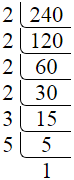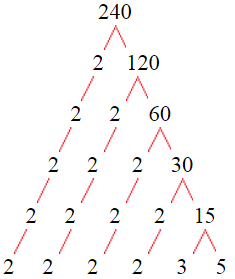# Factors of 240The factors of 240 are 1, 2, 3, 4, 5, 6, 8, 10, 12, 15, 16, 20, 24, 30, 40, 48, 60, 80, 120, and 240 i.e. F240 = {1, 2, 3, 4, 5, 6, 8, 10, 12, 15, 16, 20, 24, 30, 40, 48, 60, 80, 120, 240}. The factors of 240 are all the numbers that can divide 240 without leaving a remainder.

We can check if these numbers are factors of 240 by dividing 240 by each of them. If the result is a whole number, then the number is a factor of 240. Let's do this for each of the numbers listed above:

·        1 is a factor of 240 because 240 divided by 1 is 240.

·        2 is a factor of 240 because 240 divided by 2 is 120.

·        3 is a factor of 240 because 240 divided by 3 is 80.

·        4 is a factor of 240 because 240 divided by 4 is 60.

·        5 is a factor of 240 because 240 divided by 5 is 48.

·        6 is a factor of 240 because 240 divided by 6 is 40.

·        8 is a factor of 240 because 240 divided by 8 is 30.

·        10 is a factor of 240 because 240 divided by 10 is 24.

·        12 is a factor of 240 because 240 divided by 12 is 20.

·        15 is a factor of 240 because 240 divided by 15 is 16.

·        16 is a factor of 240 because 240 divided by 16 is 15.

·        20 is a factor of 240 because 240 divided by 20 is 12.

·        24 is a factor of 240 because 240 divided by 24 is 10.

·        30 is a factor of 240 because 240 divided by 30 is 8.

·        40 is a factor of 240 because 240 divided by 40 is 6.

·        48 is a factor of 240 because 240 divided by 48 is 5.

·        60 is a factor of 240 because 240 divided by 60 is 4.

·        80 is a factor of 240 because 240 divided by 80 is 3.

·        120 is a factor of 240 because 240 divided by 120 is 2.

·        240 is a factor of 240 because 240 divided by 240 is 1.

## How to Find Factors of 240?

1 and the number itself are the factors of every number. So, 1 and 240 are two factors of 240. To find the other factors of 240, we can start by dividing 240 by the numbers between 1 and 240. If we divide 240 by 2, we get a remainder of 0. Therefore, 2 is a factor of 240. If we divide 240 by 3, we get a remainder of 0. Therefore, 3 is also a factor of 240.

Next, we can check if 4 is a factor of 240. If we divide 240 by 4, we get a remainder of 0. Therefore, 4 is also a factor of 240. We can continue this process for all the possible factors of 240.

Through this process, we can find that the factors of 240 are 1, 2, 3, 4, 5, 6, 8, 10, 12, 15, 16, 20, 24, 30, 40, 48, 60, 80, 120, and 240. These are the only numbers that can divide 240 without leaving a remainder.

********************

********************

## Properties of the Factors of 240

The factors of 240 have some interesting properties. One of the properties is that the sum of the factors of 240 is equal to 744. We can see this by adding all the factors of 240 together:

1 + 2 + 3 + 4 + 5 + 6 + 8 + 10 + 12 + 15 + 16 + 20 + 24 + 30 + 40 + 48 + 60 + 80 + 120 + 240 = 744

Another property of the factors of 240 is that the prime factors of 240 are 2, 3, and 5 only.

## Applications of the Factors of 240

The factors of 240 have several applications in mathematics. One of the applications is in finding the highest common factor (HCF) of two or more numbers. The HCF is the largest factor that two or more numbers have in common. For example, to find the HCF of 240 and 84, we need to find the factors of both numbers and identify the largest factor they have in common. The factors of 240 are 1, 2, 3, 4, 5, 6, 8, 10, 12, 15, 16, 20, 24, 30, 40, 48, 60, 80, 120, and 240. The factors of 84 are 1, 2, 3, 4, 6, 7, 12, 14, 21, 28, 42, and 84. The largest factor that they have in common is 12. Therefore, the HCF of 240 and 84 is 12.

Another application of the factors of 240 is in prime factorization. Prime factorization is the process of expressing a number as the product of its prime factors. The prime factors of 240 are 2, 3, and 5 since these are the only prime numbers that can divide 240 without leaving a remainder. Therefore, we can express 240 as:

240 = 2 × 2 × 2 × 2 × 3 × 5

We can do prime factorization by division and factor tree method also. Here is the prime factorization of 240 by division method,240 = 2 × 2 × 2 × 2 × 3 × 5

Here is the prime factorization of 240 by the factor tree method,240 = 2 × 2 × 2 × 2 × 3 × 5

## Conclusion

The factors of 240 are the numbers that can divide 240 without leaving a remainder. The factors of 240 are 1, 2, 3, 4, 5, 6, 8, 10, 12, 15, 16, 20, 24, 30, 40, 48, 60, 80, 120, and 240. The factors of 240 have some interesting properties, such as having a sum of 744. The factors of 240 have several applications in mathematics, such as finding the highest common factor and prime factorization.